## Wednesday, December 27, 2017

Unit – 3: Production and Costs
Part: A - Production Function

1. What do you mean by production function?
Ans: Production function means the relationship between input and output which shows the maximum possible output to be produced from a given quantity of inputs.
2. What are the fixed factors of production?
Ans: Fixed factors of production are those factors of production the application of which does not change with change in output.
3. What are the variable factors of production?
Ans: Variable factors of production are those factors of production the application of which changes with the change in output.
4. What do you mean by law of increasing returns? Explain its causes.
Ans. Law of increasing returns states that when with the increase in the number of inputs, output increases more then proportionately leading to an increase in the marginal product.
Increasing returns occur owing to following reasons:
1. Greater application of the variable factors ensures better utilization of the fixed factor.
2. Greater application of the variable factor facilitates better division of labour, and
3. Greater application of the variable factor improves co-ordination between the factors.
Ans: Return to a factor refers to the behaviour of physical output owing to change in physical input of a variable factor, fixed factors remaining constant.
6. What is meant by Return to scale? Explain the various returns to scale. 2016
Ans: Returns to scale relates to long run production function or input output relation where all inputs increase at a same proportion. There are three types of return to scale.
1. Constant returns to scale,
2. Increasing returns to scale and
3. Diminishing returns to scale.
Constant returns to scale refers when the factors of production are changed in a certain proportion and output also exactly in the same proportion.
Increasing returns to scale refers when the factors of production are changed in a certain proportion and the output changes more than the rate of changes in factors.
Diminishing returns refers when the factors are changed in a certain proportion and the output changes less than the rate of change in factors.
7. Distinguish between returns to factors and returns to scale. 2016
Ans: Following are the differences between returns to factors and returns to scale.
1. Returns to factor related to the behaviour of total output when only input is increased and all other inputs are held constant. On the other hand, returns to scale refers to the increase in output when all inputs are increased in the same proportion.
2. Returns to a factor related in the short run. On the other hand, returns to scale relates in the long run.
3. Returns to a factor can be explained with the help of law of variable proportion from where we derive three stages of production function like increasing marginal return, diminishing marginal return and negative marginal returns. On the other hand returns to scale may be increasing, decreasing and constant returns.
8. What are the assumptions of the law of Returns to scale?
Ans: Following are the assumptions of the law of returns to scale:
1. There is no technological change.
2. The worker does not work without implements.
3. Perfect competition prevails in the market.
9. When is TP maximum in relation to MP?
Ans: TP is maximum when MP is zero.
10. When TP increases at increasing rate, what happens to MP?
Ans: When TP increases at increasing rate, MP should be increasing.
11. When TP increases at diminishing rate, what happens to MP?
Ans: When TP increases at diminishing rate, MP should be decreasing.
12. When TP becomes constant at its maximum, what happens to MP?
Ans: When TP becomes constant, MP should be zero.
13. Define Total Product?
Ans: It is the sum total of output produced by all units of labour (along with other factors of production).
14. Define marginal product. Or Define marginal physical product.
Ans: Marginal product or marginal physical product is the change in total product as a result of a unit change in the input of a variable factor.
15. What is meant by average product?
Ans: It is production divided by units of the variable factor (labour).
16. List any three inputs used in production.
Ans. Land, labour and capital
17. Give meaning of Cost. Or What does cost mean in Economics?
Ans. Cost is the expenditure incurred by the producer (explicitly or implicitly) on factor as well as non-factor inputs for a given amount of output of a commodity.
18. What is meant my explicit and implicit cost?
Ans. Explicit costs are those cash payments which a firm makes to others for the purchase of inputs. Example: (i) wages paid to labourers, (ii) payment made for the purchase of raw material.
Implicit costs are imputed (estimated) costs of self-owned and self-employed resources. Example: (i) interest on entrepreneur’s own capital, (ii) rent on entrepreneur’s own land used in business.
19. Define opportunity cost.
Ans. Opportunity cost is the total sacrifice made for availing an opportunity (or producing a given level of output).
20. What are selling costs?
Ans. Selling costs refer to the expenditure incurred by the producer in order to promote the sale of the commodity. Example: Expenditure on advertisement.
21. Define production cost.
Ans. Production cost refers to the expenditure incurred by the producer (explicitly or implicitly) on the inputs for producing a given level of output.
22. What is meant by fixed cost?
Ans. Fixed cost is the cost (or expenditure) incurred on the purchase (or hiring) of fixed inputs of production, like plant and machinery.
23. What is meant by variable cost?
Ans. Variable cost is the cost (or expenditure) incurred on the purchase of variable inputs of production like raw material.
24. Define total cost.
Ans. Total cost refers to all expenses incurred by the producer (explicitly or implicitly) to produce a given quantity of output.
25. What is meant by average cost?
Ans. Average cost is the expenditure incurred by the firm on the fixed factors, estimated per unit of output.
26. What is marginal cost?
Ans. Marginal cost is the change in total cost when an additional unit of output is produced.
27. State the formula to determine average cost.
Ans. Average CostOr  Average Cost = Average Fixed Cost + Average Variable Cost.
28. If the total cost of producing 5 units of a commodity is Rs. 20 and that of producing 4 units is Rs. 15, what will be the marginal cost?
Ans.29. The cost of zero level of output is equal to which cost?
Ans. The cost of zero level of output is equal to fixed cost.
30. Why does variable cost curve originate from the point of origin?
Ans. Variable cost curve originates from the point of origin because when output is zero, variable cost is also zero.
31. In which cost will the expenditure on raw material and wages of casual labourers be included?
Ans. The expenditure on raw material and wages of casual labourers will be included in the variable cost.
32. Why is total fixed cost curve parallel to X-axis?
Ans. Total fixed cost remains constant at all levels of output. So, total fixed cost curve is parallel to X-axis.
33. Why are total cost curve and variable cost curve parallel to each other?
Ans. Total cost curve and variable cost curve are parallel to each other because the difference between TC and TVC is equal to TFC which is constant at all levels of output.
34. Does average fixed cost all as output rises?
Ans. Yes, average fixed cost falls as output rises because total fixed cost is constant at all levels of output.
35. What is the general shape of AC curve?
Ans. AC curve is general ‘U’ shaped.
36. What is the general shape of MC curve?
Ans. MC curve is generally ‘U’ shaped.
37. What would you say about the average cost of a firm if the marginal cost of production is less than the average cost?
Ans. If the marginal cost (MC) of production is less than the average cost (AC), AC would be falling.
38. Why does marginal cost curve cut average cost curve only at its minimum point?
Ans. Marginal cost curve cuts average cost curve only at its minimum point because it is only here that MC = AC.
39. Define revenue.
Ans. Revenue refers to the money receipts of a firm from the sale of its output.
40. Define total revenue.
Ans. Total revenue is the sum total money receipts by a firm from the sale of its total output.
41. Define average revenue.
Ans. Average revenue is the per unit revenue corresponding to a given level of output of a firm (AR = P).
42. What is meant by marginal revenue? 2014
Ans. Marginal revenue is the addition to total revenue on account of sale of one more unit of output.
43. Which concept of revenue is called price?
Ans. Average revenue is called price.
44. Define price line.
Ans. Price line or firm’s demand curve is the same as firm’s AR curve, because AR means price, and demand curve (or AR curve) shows the relationship between price and quantity demanded of firm’s output.
45. What is the shape of total revenue curve under perfect competition?
Ans. Under perfect competition, total revenue curve is an upward sloping straight line starting from the origin.
46. What is the relation between marginal revenue and average revenue under perfect competition?
Ans. Under perfect competition, marginal revenue is equal to average revenue.
47. What are the shapes of marginal revenue curve and average revenue curve under perfect competition?
Ans. Under perfect competition, both marginal revenue curve and average revenue curve are equal and indicated by the same horizontal straight line parallel to X-axis.
48. What are the shapes of average revenue and marginal revenue curves under monopoly and monopolistic competition?
Ans. Average revenue and marginal revenue curves under monopoly and monopolistic competition slope downward from left to right. However, AR is more elastic under monopolistic competition than under monopoly.
49. Why do the marginal revenue and average revenue curves look alike under monopoly and monopolistic competition?
Ans. The marginal revenue and average revenue curves look alike under monopoly and monopolistic competition because in both the market situations, more can be sold only by lowering the price of the product.
50. When average revenue is constant, what is the shape of marginal revenue?
Ans. When average revenue is constant, marginal revenue = average revenue. Both AR and MR are indicated by the same horizontal straight line parallel to X-axis.
51. Explain the relationship between AR and MR. Give an illustration.
Ans. The relationship between AR and MR are as follows:-

1. When average revenue is constant, it is equal to marginal revenue, as under perfect competition.
2. When average revenue is diminishing, it is greater than marginal revenue. It is true in situations of monopoly and monopolistic competition.
3. Marginal revenue can be zero or negative but not the average revenue.
52. What is law of variable proportion or law of diminishing return? Mention its reasons. 2013, 2014, 2015
Ans. Law of variable proportions states that as more and more units of a variable factor are employed with fixed factors, total product increases at an increasing rate in the beginning then increases at a diminishing rate and finally starts falling.
Diminishing returns to a factor operate due to: -
1. Fixity of the factors: As more and more units of a variable factors are combined with the fixed factors, the latter gets over utilized hence the diminishing returns.
2. Factors of productions are imperfect substitute of each other.
3. The combination between gets distorted so that marginal product of the variable factor declines.
53. Give two example of variable cost. 2013, 2016
Ans. Examples of variable costs are – labour cost and costs of raw materials.
54. Give two example of fixed cost. 2014
Ans. Examples of fixed costs are – wages to permanent staff, Rent for factory building.
55. What is an iso quant curve? Explain its properties.
Ans. A curve indicates the same quantity of production of the firm. It shows a set of all possible combinations of the two inputs that would yield the same maximum possible output.
Properties or Features of Isoquant
The following are the important properties of isoquants:
1. Isoquant is downward sloping to the right. This means that if more of one factor is used less of the other is needed for producing the same output.
2. A higher isoquant represents larger output.
3. No isoquants intersect or touch each other. If so it will mean that there will be a common point on the two curves. This further means that same amount of labour and capital can produce the two levels of output which is meaningless.
4. Isoquants need not be parallel to each other. It so happens because the rate of substitution in different isoquant schedules need not necessarily be equal. Usually they are found different and therefore, isoquants may not be parallel.
5. Isoquant is convex to the origin. This implies that the slope of the isoquant diminishes from left to right along the curve. This is because of the operation of the principle of diminishing marginal rate of technical substitution.
56. Why Isoquant is negatively sloped? 2014
Ans: An Isoquant Slopes Downward from Left to Right: This implies that the Isoquant is a negatively sloped curve. This is because when the quantify of factor K (capital) is increased, the quantity of L (labor) must be reduced so as to keep the same level of output.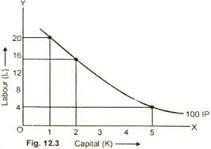56. In the long run, there is no fixed cost.  Ans. Correct
56. What is Average Product?
Ans. Average product is the per unit of product of a variable factor.56. What is marginal product?
Ans. Marginal product is an addition to the total production when an additional unit of a variable input is employed.
57. What is cost function?
Ans. Cost function shows the relationship of production cost and the physical output. The equation for cost function is given by –. Other things remaining equal and where58. Fill in the blanks: TC = ____ + TFC 2012
Ans. TC = TVC + TFC.
59. What do you mean by average variable cost?
Ans. Average variable cost is obtained by dividing the total variable cost by the number of units produced. That means,.
60. If production is zero, then give the definition of marginal cost.
Ans. If production is zero, then marginal cost is excluded.
61. Fill in the blanks – AC = AVC + ____. 2016
Ans. AC = AVC + AFC.
62. In the production function, consumer combines the factors and produce goods.
Ans. Incorrect
63. Profit is the income earned by a firm.
Ans. Incorrect
64. Production function shows the relationship between physical inputs and physical output of a firm.
Ans. Correct
65. Other factors unchanged, if one additional unit of variable factor is employed to increase production. Then it is called marginal production/total production/average production.
Ans. Marginal Production
66. What is the general shape of a marginal product curve?
Ans. Opposite U shaped.
67. What will you say about MPP of a factor when TPP rises at an increasing rate?
Ans. MPP rises.
68. When TPP is maximum what can we say about MPP?
Ans. MPP is zero.
69. When MPP is falling positive, at what rate TPP is changing?
Ans. TPP rises at diminishing rate.
70. When APP falls, what is the relation between MPP and APP?
Ans. MPP < APP
71. When APP rises, what is the relation between MPP and APP?
Ans. MPP > APP>
72. When both APP and MPP falls, what is the relation between the two’s?
Ans. APP > MPP
73. What is short period?
Ans. Short period is refer short time in which some inputs are variable and some are fixed. In this period a firm can changes only in the variable factors and not in the fixed factors.

74. What is long period?
Ans. Long period is refer to a long time in which a firm can change all factors including machinery, building etc. There is no difference between fixed cost and variable cost in the long period.
75. What does the ISO cost line show?
Ans. The ISO cost line shows two things –
1. The prices of the two factors.
2. The total outlay of the firm.
76. What is ISO revenue line?
Ans. The ISO revenue line may be defined as a straight line curve which indicates the various output combination that yield equal revenue to the firm.
77. What is marginal physical productivity curve? (MPPC)
Ans. When the firm combines a fixed amount of capital with varying units of labour, the marginal product of labour diminishes. It indicates the change in the marginal physical productivity of a variable factor as the amount of it, which is added to a fixed factor, changes.
78. Write the difference between Accounting cost and Economic cost.
Ans. Following are the difference between accounting cost and economic costs are as follows:
1. Accounting costs relates to those costs only which involve cash payments by the entrepreneur. On the other hand economic costs take into account these accounting costs but they also include the amount of money the entrepreneur could have earned if he had invested his money and sold his own services.
2. Accounting costs are also called explicit costs whereas the economic costs include both accounting costs and implicit costs.
79. What are the major components of total costs?
Ans. The major components of total costs are:
1. Material cost.
2. Wages.
3. Other cost.
80. What are the determinants of costs?
Ans. The following are the determinants of cost behaviour:
1. Law of returns.
2. Size of the plant.
3. Period under consideration.
4. Level of capacity utilization.
5. Prices of factors of production.
81. What is the other name of fixed cost?
82. Why does it call overhead cost?
Ans. The fixed costs are referred to as overhead costs because these costs are common to all the unit of the commodity produced and not special to any one of them.
83. Why does the average variable cost per unit increase so sharply after the normal capacity?
Ans. The reason is that as the output increases beyond the normal capacity at the point M through the employment of more and more workers, it leads to overcrowding and bad organization in the factory. This result is that the variable costs per unit go on increasing with every increase in the output.
84. What does the AFC curve look like? Why does it look so? 2016
Ans. AFC curve is downward sloping to right. As the output increases, the average fixed costs get distributed that is AFC falls.
85. Can the average cost be more than the marginal cost, when the average cost is falling?
Ans. Yes.
86. Give the meaning of constant returns.
Ans. The law of constant returns means when all factors of production are increased in given proportion the product or output also increase in the same proportion.
87. Give the meaning of economies of scale.
Ans. It implies extra benefits enjoyed by a firm or industry as a whole because of more expansion in the scale of production.
88. What are the types of Economies of scale?
Ans. The two types of economies of scale are –
1. Internal economies.
2. External economies.
89. What is an internal economy?
Ans. Internal economies are those economies which arises within a firm and therefore, internal to the firm. They may arise due to expansion of a firm and are not shared by other firms in the industry.
90. What is an external economy?
Ans. External economies are those economies which arise as a result of expansion of the industry due to larger size of an industry.
91. What are the assumptions of least cost combination?
Ans. Following are the assumptions of least cost combination:
1. Perfect competition prevails in the input market.
2. The market price of inputs are given and fixed.
3. The marginal product of every input in self-dependent, that is not dependent on the other.
92. What is Cobb-Douglas Production function?
Ans. It refers the technological relationship between inputs and output for the entire output production. In the Cobb-Douglas production function
93. What are the reasons for the Downward Sloping Demand Curve?
Ans. (a) The Law of Diminishing Marginal Utility
(b) Law of Equi-Marginal Utility.
(c) Income Effect.
(d) Substitution Effect.
94. What is cross price effect? Give two examples to illustrate it.
Ans. Cross Price Effect: When demand for a particular product is affected by change in the price of another product, it is called cross price effect. It originates from related goods like substitute goods and complementary goods. For example, if price of coffee falls, then quantity demanded of its substitute tea will fall because consumers will substitute coffee for tea. This shows cross price effect since change in price of one product (coffee) has affected demand for other product (tea). Similarly a fall in price of complementary good like petrol increases the demand for its complementary good car or bike.
B. Questions for Marks – 3/4
1. Distinguish between input or output?
Ans. Distinctions between Input or Output are given below –
1. Input may be used to produce a goods or services. On the other hand, output means creation of utility.
2. Input is essential to produce output. On the other hand, it may not be always possible that using inputs creates output.
3. The inputs are raw-materials. But the outputs are final goods.
2. Distinguish between fixed input and variable input?
Ans. Distinctions between fixed input and variable input are given below –
1. The fixed inputs are that input which does not vary in the short period. On the other hand, variable inputs are those which vary both in short or long period.
2. Fixed inputs for example, machinery, and salary of permanent employees. On the other hand variable inputs for example, raw materials, wages of labour etc.
3. Fixed input and variable input are differentiated only in the short period. But there is no distinction between the two in the long period.
3. Write the difference between short period and long period? 2014
Ans. Distinctions between short period and in long period are given below –
1. Short period is such type of period in which producers does not change all the factors. But on the other hand, long period is such type of period in which producers changes all the factors.
2. In the short period, producer changes its production only by changing variable factor. On the other hand, in the long period, a producer changes its production both by fixed and variable factors.
3. In the short period demand plays an active role for determining price of the commodity. On the other hand, in the long period both demand and supply determine price of the commodity.
4. Draw and explain the shape of the average variable cost curve.
Ans. AVC is obtained by dividing the total variable cost by the quantity of output. AVC curve is drawn and explain the shape of the curve in below.
In the diagram, OX-axis represents quantity of output and OY-axis represents average variable cost. At zero level of production total variable is zero. As soon as production increases, the average variable cost declines. In the diagram, the average variable cost and declined up to OQ, level of output and after that it goes on increasing with the increase in output.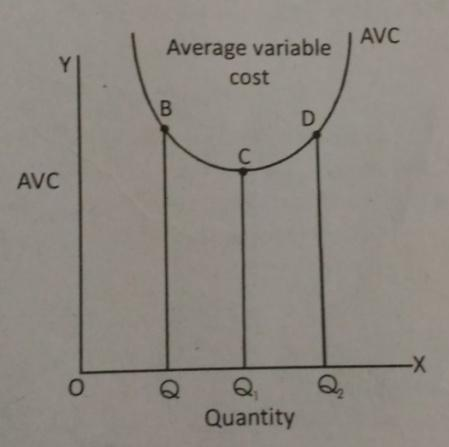In the above diagram, it is shown that when production increases from OQ1 and OQ2, the average variable cost rises from OQ1 to OQ2. So, the average variable cost curve is U-shaped. Because as production increases the average variable cost is also increases.
5. Show with the help of a diagram that the total cost is the vertical sum of total fixed cost and total variable cost. 2012
Ans: Relationship between TC, TFC and TVC: Total fixed costs are those which do not vary with different levels of production. For example, the cost of machinery, salary of permanent staff, rent of the land etc. The cost that a producer incurs to employ these fixed inputs is called the total fixed cost (TFC). Total variable costs are those, which vary almost in direct proportion to volume of production. That means, when production is increase average variable cost is also increases, and vice-versa. For example, wages of labour, raw materials etc. Total cost is the sum total of total fixed cost and total variable cost. That means TC = TFC + TVC.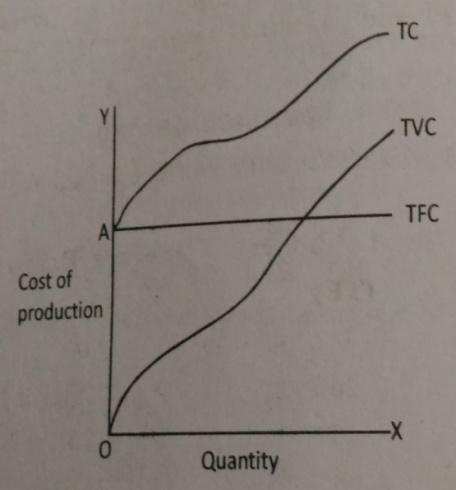In the diagram, OX-axis represents cost of production and OY-axis represents quantity. Fixed cost remains unchanged for any level of output. So the TFC curve is parallel to the OX-axis. If the production is zero, total variable cost is also zero. Hence the point of origin of the total variable cost is zero. As the total cost curve is the sum total of TVC and TFC. So the total cost is start from point “A”.
6. What are Relationship between AP and MP? 2012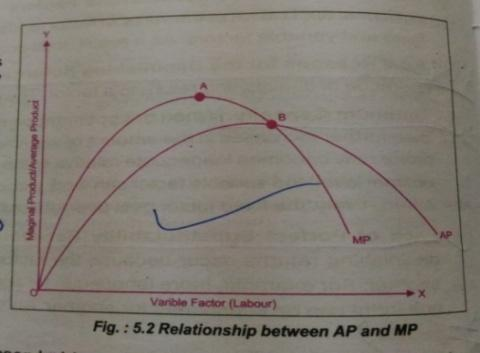Ans. The relationship between the two is as follows –
1. As we increase the amount of an input, MP rises AP being the average of marginal products, also rises, but rises less than MP.
2. The average product curve slopes upwards as long as the marginal product curve is above it. It makes no difference whether the MP curve itself is sloping upwards or downwards.
3. When MP falls sufficiently, it becomes less than the prevailing AP and the later (i.e. AP) also starts falling. So MP and AP both are inversely U shaped.
4. The slope of the AP curve depends on whether MP is above or below AP. When MP is above AP, the AP curve slopes upwards and when MP is below AP, the AP curve slopes downwards.
5. MP can be zero and negative but AP is always positive.
7. Explain the relationship between the marginal product and the total product of an input. 2015
Ans. The relationship between the marginal product and the total product of an input are given below –
1. When marginal product increases, total product increases at an increasing rate.
2. When marginal product falls but remains positive total product increases at a decreasing rate.
3. If marginal product is constant over a range of production, it implies that the total product increases at a constant rate.
4. If marginal product is negative, then total product falls.
8. Explain the relationship between ATC, AVC, AFC and MC.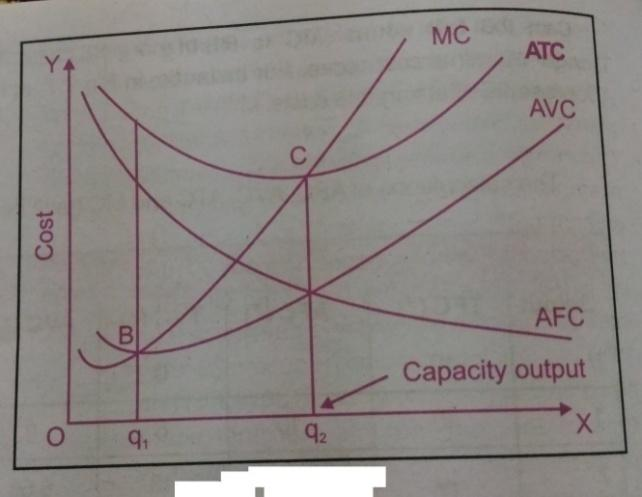Ans. Initially as output increases, AVC and ATC fall and then rise as output increases. The distance between the ATC curve and the AVC curve gets smaller as output increases. AFC is a high percentage of ATC at early levels of output and low percentage of ATC as output increases. Alternatively speaking, AVC is a low percentage of ATC at early levels of output and high percentage of ATC at higher levels of output. This is why at higher levels of output ATC comes closer to AVC. However, ATC and AVC curves never intersect each other. Since AFC can never be zero or negative. ATC will always be greater than AVC. Marginal cost curve like ATC and AVC curves falls in the beginning then it intersects the AVC and ATC curves at their minimum point B and C respectively. Q2 is the capacity output where ATC curve reaches the minimum point. MC is less than the AC when AC is falling and more than the AC when AC is rising. Therefore MC curve cuts the AC curve from below at the minimum point of AC.
9. Explain the differences between explicit costs and implicit costs. 2015
Ans. The differences between explicit costs and implicit costs are as follows –
 Basis Explicit Costs Implicit Costs 1. Meaning There are the costs of hired/purchased inputs. These are the costs of self supplied inputs which are used in production. 2. Payment Explicit costs are the cost of inputs whose payment is made to the out orders. Implicit costs involve no money payment. These include the imputed value of self inputs supplied. 3. Examples (i) Payment of wages. (ii) Payment of rent of hired building. (iii) Payment for raw material purchased. (i) Interest on own capital. (ii) Rent of own land and building. (iii) Estimated salary of the owner who is acting as a manager.
10. Distinguish between fixed cost and variable cost and give one example of each.
Ans. The differences between fixed cost and variable cost are discussed below –
 Fixed Costs Variable Costs 1. Fixed costs are fixed and do not change with change in output e.g. expenditure on machines, wages paid to payment staff. 1. Variable costs change with level of output e.g. expenditure of raw material, payment of electricity bill. 2. Fixed costs can never be zero. They remain the same at all levels of output. 2. Variable cost is zero when production is stopped.
11. Why is the MC curve U shaped?
Ans. The marginal cost curve is U-Shaped. The shape of MC curve is governed by the law of variable proportions. Initially as employment of the variable factor increases, the marginal product of the factor increases. Then after a certain point, it decreases. As a result, initially MC falls and after a point it rises. Therefore MC curve is U shaped.
12. Explain the relationship between AVC and AFC? 2013

Ans. AVC curve is U-shaped as it falls upto a point and then start rising. The law of variable proportions explains why AVC is U-shaped whereas AFC curve is a rectangular hyperbola. AFC always decreases with increase in the level of output. It is because TFC remains constant at all levels of output. But AVC initially falls and then starts rising. As a result, the difference (or gap) between AC and AFC increases with increase in the level of output
13. What is the general relationship between AR and MR?
Ans. The relationship between AR and MR are discussed below –
1. AR increases as long as MR is higher than AR.
2. AR remains constant when MR is equal to AR.
3. AR falls when MR is less than AR.
14. What is the relationship between average cost and marginal cost? Use diagram. 2014
Ans. Relationship between AC and MC
We observe the following relationship between AC and MC is –
1. Both average cost and marginal cost are obtained from total cost as under:1. When average cost falls with an increase in output (upto q, level of output as shown marginal cost always remains lower than average cost.
2. When average cost is rising, marginal cost remains above average cost. In other words, marginal cost rises faster than average cost (beyond q1 level of output).
3. Marginal cost and average cost are equal when average cost in minimum (at q1 level of output).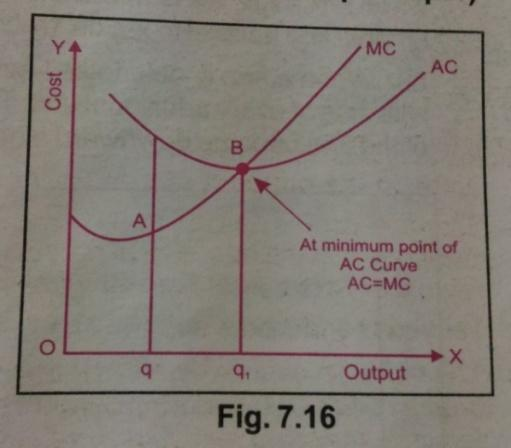15. Write the short notes on short run production function.
Ans. The law of variable proportion relates to the short run production function. In the short run production function, there are two factors, labour and capital where capital is fixed and labour is variable.
This production function can be expressed as: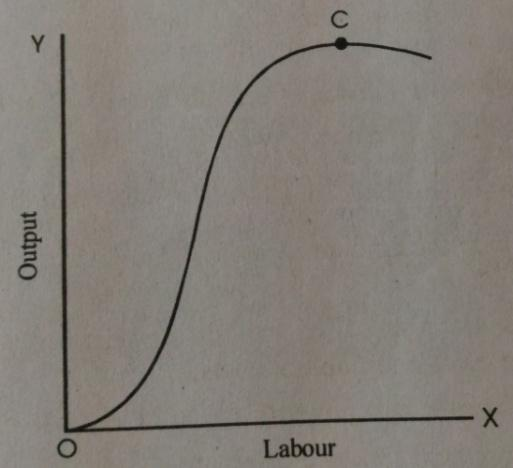The following diagram shows the short run production function.
Initial output increases more than in proportion to labour, so that the average product of labour rises. As the number of workers rises further, output may not increase as much per worker total output increases less then in proportion to labour, so the average product falls. The marginal product of labour also declines. Production beyond point c is not part of the production function, because no firm would produce with that many workers.
16. Why does the U-shaped of the long run cost curves is less pronounced compared with U-shape of the short run cost curves?
Ans. LAC curve will be U-shape like the short run cost curves but its U-shape will be less pronounced than that of the short run cost curves. It will be flatter. In the short period, some of the factors of production, such as machinery and management are indivisible. They lack flexibility and cannot be used in differing proportions to bring about changes in output. But in the long run, these indivisible factors become to some extent, divisible. Because, in the long period, availability of adequate time, the size of these factors (machinery, management) can be altered in different proportions for the purpose of production. That means in the long period, the size of its firm can be increased to deal more economically in its increased output.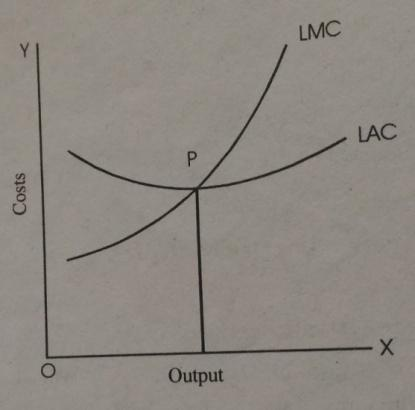17. Explain producer’s equilibrium in the long run.  Or Why does a firm earns a normal profit in a long run?
Ans. In the long period, new firms can freely enter the market (industry) or the existing firms can leave the industry. Due to the sufficient time period, there is free entry and exit of firms in the market. Due to this reason, market price will be equal to average cost of production and firms are making only normal profits. The level of output at which a producer earns only normal profit is called the breakeven point. This situation has been shown in fig.
Producer (under perfect competition) will be in long period equilibrium at a point where there are neither abnormal profits nor abnormal losses. Here E is the equilibrium and OQ the equilibrium level of output. At OQ level of output LMC = LAC = MR.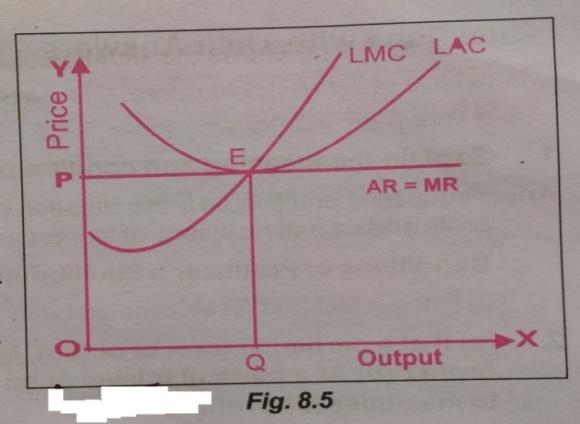In the long period all costs are variable which have to be covered by all means. Therefore, in the situation or abnormal losses, some existing firms (inefficient or marginal firms) will start leaving the industry. Fall in the number of firms in the industry would then imply less output (or supply). Fall in industry’s output will raise the product price in the market.
18. Explain the concept of producer’s equilibrium for:
1. Abnormal profit.
2. Normal profit.
Ans. In the short period, neither firms can enter nor leave the industry. Accordingly, the number of firms producing a commodity remains fixed in the short run. In the short period, a producer (under perfect competition) may earn abnormal profits (also called super normal profits) or normal profits. I may also have to bear even losses (abnormal losses). A competitive firm may able to earn super normal profits when market price of the product (as determined by the industry) is greater than the average cost (i.e. P > AC). And the producer will have to bear loss when market price is lower than the average cost of production (i.e. P < AC). The producer will earn normal profits when price is equal to average cost (i.e. P = AC). These situations have been illustrated diagrammatically as under:
1. Shows the situation of abnormal profits. The producer will be in equilibrium at point E (where MC = MR). Therefore, OQ will be the profit maximizing output for the producer. At this level of output (i.e. OQ), average cost is QC which is lower than the market price QE (=OP). Producer is earning CE (price minus average cost) per unit profit. Total profits are given by the area BPEC (per unit profit x quantity of output). These are super normal profits which are over and above the total costs. Remember, total costs include normal profits.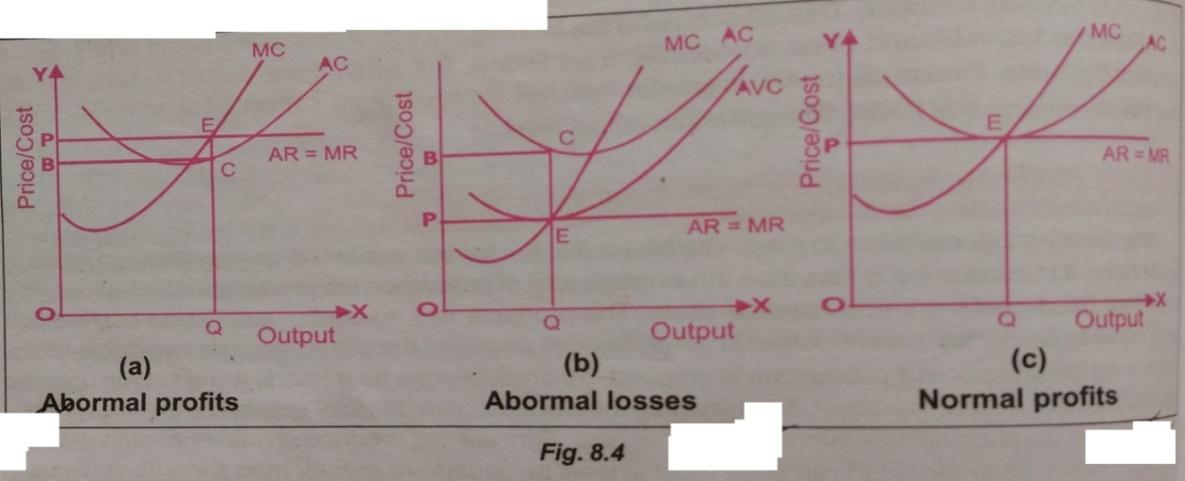1. Shows the situation of abnormal losses. Here E is the equilibrium point where MC = MR and OQ is the equilibrium output. At this level of output, price QE (=OP) is lower than level of average cost which is QC. Firm is here incurring losses as P < AC. CE is the loss per unit of output. Total losses amount to area PBCE (CE x OQ). Hence, in the short run, there are three conditions of equilibrium, (i) MC = MR (ii) MC must be rising and (iii) PAVC.
2. Shows the situation of normal profits. Here market price (OP) is equal to producer’s average cost of production (QE) at equilibrium level of output. OQ is the equilibrium level of output as MC curve intersects MR (=AR) curve at this level of output. At OQ level of input MC = MR = Price. Abnormal profits or loss is here nil.
Part B: Supply Theory
1. What do you mean by supply?
Ans. Supply means the amount offered for sale at a definite price during a certain period of time.
2. What is individual supply?
Ans. An individual supply means a schedule of an individual seller of a commodity which is supplied in the market in a definite price during a certain period.
3. What is supply curve?
Ans. The supply curve depicts the relationship between price of the commodity and its quantity. This relationship is direct. It is obtained from the supply schedule.
4. What is market supply?
Ans. Market supply is the aggregate supply that is the supply offered by all the firms put together.
5. What is supply schedule?
Ans. Supply schedule shows a price schedule which shows the amount of supply at different prices.
6. Write the law of supply?
Ans. According to law of supply, “other things remaining constant’ the price of a commodity rises the supply is extended and as the price falls its supply is contracted.
7. Name one commodity whose supply is inelastic.
Ans. Salt.
8. Mention one factor which determines supply of a commodity.
Ans. Improvement in techniques of production
9. What indicates of vertical supply curve? 2013
Ans. Vertical supply curve indicates perfectly inelastic supply. It means with changes in price there is no change in supply.
10. What is the value of vertical supply curve?
Ans. Zero.
11. What do you mean by change in supply?
Ans. Change in supply means change in quantity supplied (rise or fall) as the price changes.
12. What is excess supply?
Ans. When the market price of the commodity is higher than the equilibrium price, then at the price quantity supplied exceeds quantity demanded. This is called excess supply.
13. What is excess demand?
Ans. When the price of commodity is lower than the equilibrium price, then at the price quantity demanded exceeds quantity supplied. This is called excess demand.
14. Write three factors which determine the elasticity of supply.
Ans. Three factors which determine the elasticity of supply are as follows –
1. Behaviour of cost of production.
2. Availability of factors of production.
3. Nature of goods.
15. Why does the market period supply curve of a perishable commodity is perfectly inelastic or vertical straight line? 2014, 2015
Ans. Market price is the price of a good which prevails at any given period. It is determined by the intersection of demand and supply at a time. The perishable goods like fish, milk etc. cannot be stored or kept back. Therefore, the whole of the given stock of a perishable good has to be supplied in the market, whatever the price of the good. As a result, the market period supply curve of a perishable commodity is perfectly inelastic or vertical straight curve of a perishable commodity is perfectly inelastic or vertical straight line.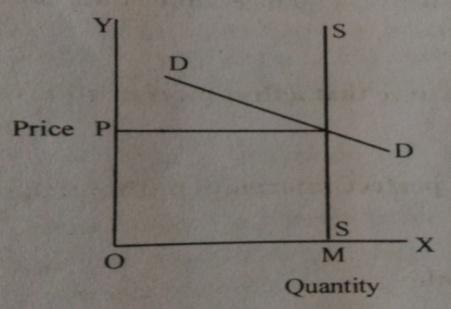16. Why does the supply curve of the industry in the short run under perfect competition is upward sloping?
Ans. Under perfect competition in the short run, supply curve is the lateral summation of the short run marginal cost curves of the firm. The supply curve of the industry in the short run under perfect competition is upward sloping because the short run marginal cost curves of the individual firms are upward sloping.
17. What is the shape of long run supply curve in the increasing cost industry?
Ans. It is upward sloping.
18. What is the shape of supply curve in case of constant cost industry?
Ans. It is horizontal.
19. What is the shape of supply curve in case of decreasing cost industry?
Ans. It is downward sloping curve.
20. Explain the long run supply curve f a firm.
Ans. The long run supply curve of a firm is explained by two-parts.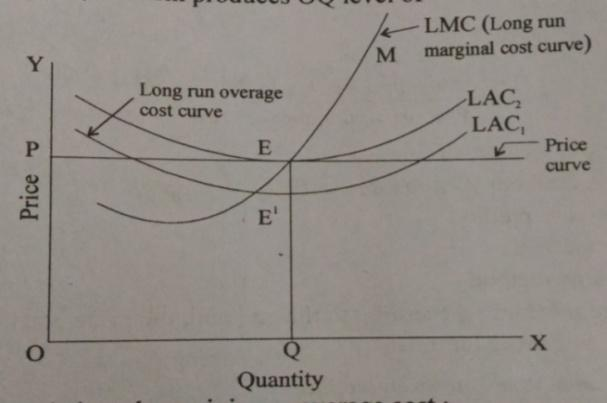1. Market price is equal or greater than minimum long run cost curve – In the diagram, it shows that market price (p) is greater than the minimum average cost (LAC) and the price curve is intersect by LMC curve at point E. At that point E, LMC and LMR or price are equal at OQ level of output. According to long run condition of profit maximization of a firm, price must be equal to minimum long run average cost or greater than average cost. This condition is fulfilled at that level of OQ amount of output. In the diagram, it is shown that at OP price, the firm produces OQ level of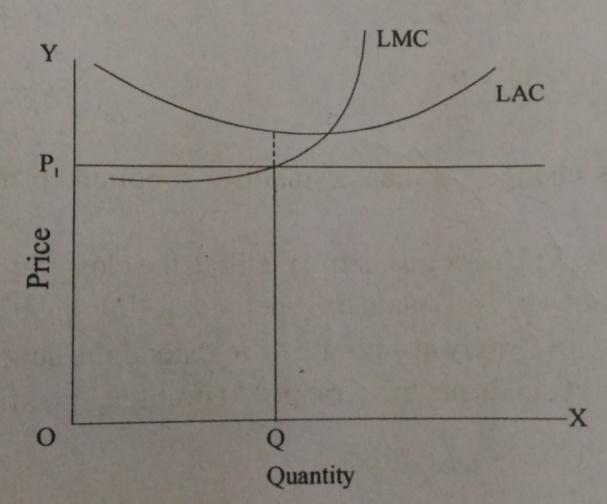2. Market Price is less than minimum average cost – If the market price (P1) is less than minimum average cost then the firm will not be able to earn positive supply of a commodity. Because, for equilibrium and maximum profit of a firm, the price is always equal or greater than long run marginal cost. But, in the diagram the market is less than average cost. So, at the price the production is zero. The long run supply curve is the rising part of the Marginal cost curve which rising upward from the minimum point of long run average cost curve. In the diagram (A) E, M part is the long run supply curve of a firm.
21. Explain the law of supply with the help of a supply schedule diagram.
Ans. Law of supply:- The law of supply states that, other things being equal, quality supplied increases with increases in price and decrease with decrease in price. In other words, there is positive relationship between price and quantity supplied.
We can explain law of supply with the help of a schedule.(Rs.)(units) 10 11 12 100 200 300
The above schedule shows that quantity supplied increases from 100 to 200 units. When own price of the commodity increases from Rs. 10 to Rs. 11 per unit. Likewise, it increases from 200 to 300 units when increases from Rs. 11 + Rs. 12 per unit. Implying that there is positive relationship between own price of the commodity and its quantity supplied.
22. What are assumptions of the law of supply?
Ans. Assumption of the law of supply are as follows:-
1. There is no change in any change in the price of the factor of production.
2. There is no in the technique of production.
3. There is no change in the goal of the firm.
4. There is no change in the price of the related goods.
5. There is no change in business expectation.
6. The government policy of relating to the price and production of the commodity does not change.
23. What are the exceptions to the law of supply?
Ans. Following are the exceptions to the law of supply are as follows:-
1. The law of supply does not apply strictly to agricultural product whose supply is governed by natural factors.
2. Supply of goods having social distinction will remain limited even if their price tends to rise.
3. At a given point of time, sellers may be willing to sell more of perishable commodity even at a lower price.
24. What is change in supply? Explain the factors that changes supply of a commodity. 2012, 2014, 2016
Or
What are the factors which bring changes in supply curve?
Or
What are the various determinants of supply.
Ans. Change in supply means the shift of supply curve. With increase in supply, supply curve shifts to the right and with decrease in supply, the supply curve shifts to the left. Following factors cause a change in supply:
1. Technological Change: When there is technological improvement, output is produced at lower cost. Lower cost of production increases the supply of the commodity. The supply curve shifts to the right.
2. Price of Other Goods: When price of a substitute good increase, the quantity supplied of the concerned good decreases and there is backward shift of the supply curve. Supply curve shifts forward in case price of the substitute good decreases.
3. Price of Factors of Production: If factor price decreases, cost of production also decreases. Accordingly supply increases and the supply curve shifts to the right. If factor price increases, supply tends to decline and there is a backward shift in supply curve.
4. Number of Firms: Increase in the number of firms implies increase in market supply and conversely decrease in number of firms reduces the market supply of the commodity.
25. What are the causes for increase in supply?
Ans. Causes for increase in supply are as follows:-
2. Reduction in factor prices, causing fall in cost of production.
3. Price substitute goods fall in the market.
4. Increase in number of firm in the industry. It causes increase in market supply even when price of the commodity remain the same.
5. High business expectation. It prompts the producers to increase investment accordingly, supply increases.
6. Shift in goal of the firm from profit maximization to sales maximization.
7. Decrease in taxation or grant of subsidy.
26. What are the causes of decrease in supply?
Ans. Decrease in supply causes due to following reasons:-
1. Use of outdated technology, causing fall in efficiency and rise in case of production.
2. Increase in factor price, cause increase in cost of production.
3. Rise in price of substitute goods.
4. Decrease in number of firm in the industry.
5. Low business expectations. It prompts the producer to reduce investment according supply reduces.
6. Shift in goal of the firm from sales maximization to profit maximization.
7. Increase in taxation or withdrawal of subsidy. It prompts the producers to restrict supply of their product.
27. Distinguish between extension of supply and contraction of supply.
Ans. The main differences between the two are as follows:-
 Extension of supply Contraction of supply (i) Extension of supply refers to increase in supply of commodity due to rise in its price. (ii) Extension of supply occurs due to increase in price. (iii) Diagrammatically it is shown by a upward movement on supply curve. (i) Contraction of supply refers to decrease in supply of commodity due to fall in price. (ii) Contraction of supply occurs due to fall in price. (iii) Diagrammatically it is shown by a downward movement on supply curve.
28. Distinguish between increase in supply and extension.
Ans. The differences between the two are as follows:-
 Increase in supply Extension  of supply (i) Increase in supply refers to increase in supply of a commodity at its existing price. (ii) Increase in supply occurs due to change in factors other than price of the commodity. (iii) Diagrammatically, it is shown by a forward shift in supply curve. (i) Extension refers to increase in supply of a commodity due to increase in its price. (ii) Extension of supply occurs due to change in price of the commodity. (iii) Diagrammatically it is shown by a upward movement on the same supply curve.
29. Distinguish between Decrease in supply and Contraction.
Ans. The differences between the two are as follows:-
 Decrease in supply Contraction of supply (i) Decrease in supply refers to decrease in supply of a commodity at its existing price. (ii) Decrease in supply occurs due to change in factors other than price of the commodity.. (iii) Diagrammatically, it is shown by a leftward shift in supply curve. (i) Contraction refers to decrease in supply of a commodity due to decrease in its price.   (ii) Contraction of supply occurs due to change in price of the commodity. (iii) Diagrammatically it is shown by a downward movement on the same supply curve.
30 .Distinguish between change in supply and change in quantity supplied. 2015

Ans: A change in supply and a change in quantity supplied are different things.  The first is shown graphically as a movement of a supply curve while the second is shown as a movement along a curve.  The first is caused by changes in costs and incentives that change how much a producer can and will produce at a given price.  The second is caused simply by a change in the retail price of the product.